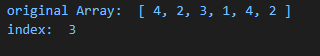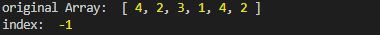Open in App
Not now

# Lodash _.findIndex() Method

• Last Updated : 14 Jul, 2020

Lodash is a module in Node.js that works on the top of Underscore.js. Lodash helps in working with arrays, strings, objects, numbers etc.
The Loadsh.findIndex() method is used to find the index of the first occurrence of the element. It is different from indexOf because it takes the predicate function that iterates through each element of the array.

Syntax:

`findIndex(array, [predicate=_.identity], fromIndex)`

Parameter: This method accepts three parameters as mentioned above and described below:

• array: It is the array in which the value is to be searched.
• predicate: It is the function that iterate through each element.
• fromIndex: It is the index after which the element is to be searched. If from index is not given by default it will be 0.

Return Value: It return the index of the element if found else -1 is returned.

Note: Please install lodash module by using command npm install lodash before using the code given below.

Example 1: When element is search start from index 0.

## Javascript

 `// Requiring the lodash library ` `const _ = require(``'lodash'``); ` ` `  `// Original array ` `let array1 = [4, 2, 3, 1, 4, 2] ` ` `  `// Using lodash.findIndex ` `let index = _.findIndex(array1, (e) => { ` `    ``return` `e == 1; ` `}, 0); ` ` `  `// Print original Array ` `console.log(``"original Array: "``, array1) ` ` `  `// Printing the index ` `console.log(``"index: "``, index) `

Output :Example 2: When element is looked after some index “i”. Here element is present in the array but still the output is -1 because it is present at index 3 and the searching starts from index 5.

## Javascript

 `// Requiring the lodash library ` `const _ = require(``'lodash'``); ` ` `  `// Original array ` `let array1 = [4, 2, 3, 1, 4, 2] ` ` `  `// Using lodash.findIndex ` `let index = _.findIndex(array1, (e) => { ` `    ``return` `e == 1; ` `}, 5); ` ` `  `// Print original Array ` `console.log(``"original Array: "``, array1) ` ` `  `// Printing the index ` `console.log(``"index: "``, index) `

Output:My Personal Notes arrow_drop_up
Related Articles